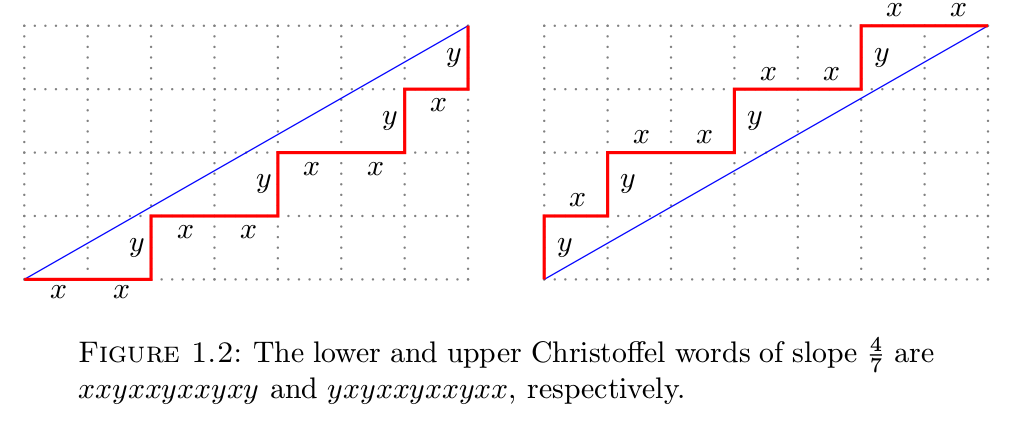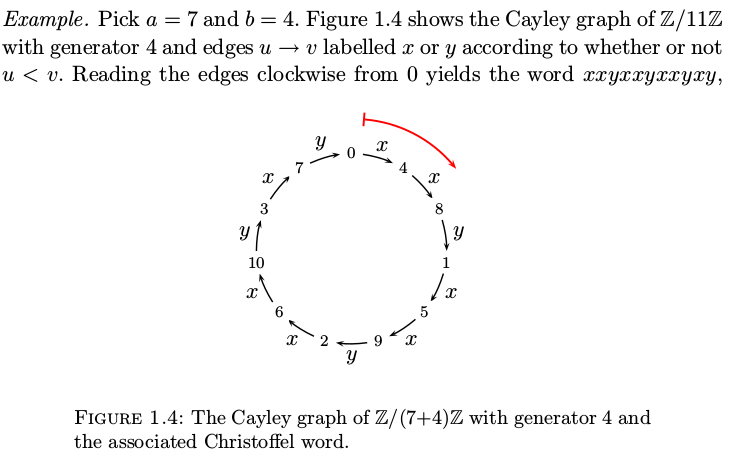## § Lyndon + Christoffel = Convex Hull

Actual "real world" use-case of lyndon factorization, cribbed from here:
• Combinatorics on Words: Christoffel Words and Repetitions in Words
I wanted to learn a real-world use-case of lyndon factorization so I could remember it. I found a whole bridge between combinatorics/strings and discrete geometry (they call it "digital geometry") that I didn't know existed before.
• If you have a line with rational slope $p/q$ and you want to draw a "discretized line" by connecting integer points in ZxZ, you can describe this discretized line as starting from $(0, 0)$, making moves $x$ (move up 1 unit along $x$-axis), $y$ (move up 1 unit along $y$-axis), finally reaching the point $(p, q)$. For example, to reach the point $(2, 3)$, you can make the moves $[x, x, x, y, y]$.
• A christoffel word is a word $w \in \{x, y \}^\star$ such that it hugs a line of rational slope $p/q$ as close as possible. Formally, there are no integer points between the line with slope $p/q$ starting from the origin, and the discretized line as described by $w$. An example picture:• It turns out that all primitive christoffel words are Lyndon words. I'm not 100% sure what primitive is, but the take-away is that these primitive christoffel words represent discrete lines that are good approximations of lines.
• Now, we are given a discrete sequence of adjacent line segments going upwards, where the line segments are described by $x, y$ moves. We want to check if the discrete curve defined by them is well-approximating a convex polygon.
• We compute the lyndon factorization of the word. This splits the original line segment into a series of line segments, where each successive line segment has lower slope than the previous (since the lyndon decomposition splits words into non-decreasing lex order).• We can then check that each word in the lyndon decomposition is a Christoffel word. If it is, then your sequence of moves describes a "good discrete convex hull", since as described above, a christoffel word "hugs the line" well.

#### § Bonus: slick characterization of line drawingIf we want to draw a line with slope p/q = 4/7 using the lower approximation the idea is that we keep taking 4 steps along x, and every time we "exceed" 7 steps along x, we take a step along y. This is the same as:
1. working in Z/(4+7)Z, starting from 0, writing down multiples of 4 till we cycle back to 0
2. marking an "increase" in a step with an x, and a "decrease" in a step with y.
The intuition is that once we walk k*p steps where k*p >= q, we want to increment y. So, at first glance, we may believe we should consider Z/qZ. However, this is misguided. Examples to enlighten:
1. Consider x=1, y=0. We should use Z/1Z = { 0 }: that is, we must keep moving along 0 -x -> 0 -x-> 0 -x-> .... This is unlike what happens if we choose Z/0Z (which is not a well-defined idea).
2. Consider x=1,y=1. We should use Z/2Z, so we keep going 0 -x-> 1 -y-> 0 -> ...which will cause is to flip x -> y -> x -> y -> ....
In some sense, we are making sure that we can "start" with an x and see where that takes us. In the Z/1Z case, we realise that we can keep taking xs. In the Z/2Z case, we realise we need to flip between x and x. Let's try to show this formally, where k is the smallest number such that kp >= q. We'll also have concrete examples where p=2, q=7. For the example, k=4 since kp = 4*2 = 8 > 7. If we work in Z/yZ = Z/7Z, we will get the numbers:
Z:    0, p, 2p, ... (k-1)p, [q] kp
Z/qZ: 0, p, 2p, ... (k-1)p, [q] (kp % q)
Z/7z: 0, 2,  4, ...      6,  (8 % 7 = 1)

But notice that we are inserting x between numbers. So we will get:
Z:    0 -x-> p -x-> 2p, ... (k-1)p -y-> (k+1)p
Z/qZ: 0 -x-> p -x-> 2p, ... (k-1)p -y-> ((k+1)p % y)
Z/7z: 0 -x-> 2 -x->  4, ...      6 -y-> (8      % 7 = 1)
^                            ^
x since [0 < 2]              y since [6 > 1]

which gives us the moves:
Z/7Z: 0 -x-> 2 -x-> 4 -x-> 6 -y-> 8 % 7 = 1

We only get 3 occurences of x, after which on the next accumulation of p, becomes an 8 which wraps around to a 1. This is the age-old tension that exists between points and gaps . For k points, there are k-1 gaps. In our case, we have 4 points [0, 2, 4, 6], but this leaves us room for only three gaps for three x moves, while in reality we need 4. We remedy this situation by giving ourselves space enough for one more x, by changing from Z/qZ to Z/(p+q)Z. We should look at this as creating space for another gap.
Z:        0, p, 2p, ... (k-1)p, [q] kp,  [q+p  ], (k+1)p
Z/(p+q)Z: 0, p, 2p, ... (k-1)p, [q] kp,  [q+p  ], (k+1)p % (p+q)
Z/9z: 0, 2,  4, ...          6,   8,  [7+2=9], (10    %    9=1)

which gives us the moves:
Z:        0 -x-> p -x-> ... (k-1)p -x-> kp -y-> (k+1)p
Z/(p+q)Z: 0 -x-> p -x-> ... (k-1)p -x-> kp -y-> (k+1)p % (p+q)
Z/9Z:     0 -x-> 2 -x-> ...      6 -x->  8 -y-> (   10 %     9 = 1)
^                             ^
x since [0 < 2]               y since [8 > 1]


That is, we are able to get k occurences x between 0, p, ..,kp which has (k+1) points. Concretely, we have:
Z/9Z: 0 -x-> 2 -x-> 4 -x-> 6 -x-> 8 -y-> 1

where we have 4 occurences of x in between after which we have an occurence of y, which is what we want when x=2, y = 7. We need to reach at least q=7 before we have exceeded our denominator and need to make a move along y.